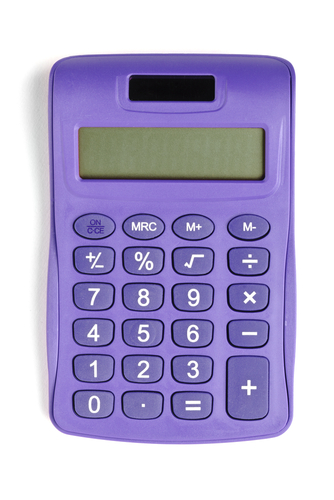# Use a Calculator to Calculate Reverse Percentages

In this worksheet, students will use a calculator to find an original quantity when they are given a percentage of that amount.Key stage:  KS 3

Curriculum topic:   Number

Curriculum subtopic:   Interpret Fractions/Percentages as Operators

Difficulty level:#### Worksheet Overview

If 73% of a quantity is 328.5, what amount did we start with?In other words, we are trying to find what 100% is.

To do this, we need a calculator.Firstly, we can turn 73% into a decimal by doing 73 ÷ 100 = 0.73

Next, because we want to go back to find what the original quantity was, we would do:

328.5 ÷ 0.73 = 450

Check:

73% of 450 = 450 x 0.73 = 328.5so we are right!

Notice how our original quantity is bigger than the 73%?

This makes sense, since we are finding what 100% is!Example 1

Use a calculator to find 100%, given that 50% is 250.

First, find our multiplier by dividing the percentage by 100.

50 ÷ 100 = 0.5

250 ÷ 0.5 = 500

So 100% of the quantity must be 500.

Example 2

If 80% of a number is 42, what is 100%?

80 ÷ 100 = 0.8

42 ÷ 0.8 = 52.5Are you ready to try some questions?

### What is EdPlace?

We're your National Curriculum aligned online education content provider helping each child succeed in English, maths and science from year 1 to GCSE. With an EdPlace account you’ll be able to track and measure progress, helping each child achieve their best. We build confidence and attainment by personalising each child’s learning at a level that suits them.

Get started